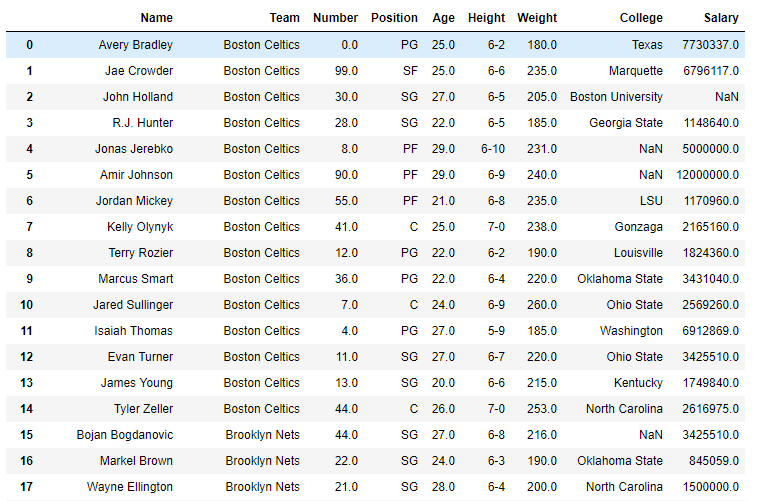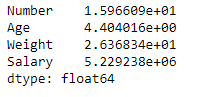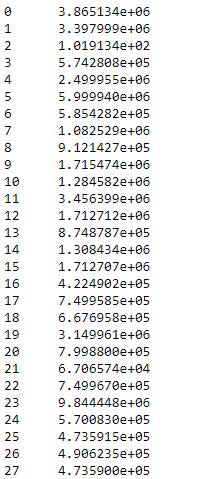Skip to content
Related Articles
Python | Pandas dataframe.std()
• Last Updated : 22 Oct, 2019

Python is a great language for doing data analysis, primarily because of the fantastic ecosystem of data-centric python packages. Pandas is one of those packages and makes importing and analyzing data much easier.

Pandas` dataframe.std()` function return sample standard deviation over requested axis. By default the standard deviations are normalized by N-1. It is a measure that is used to quantify the amount of variation or dispersion of a set of data values. For more information click here

Syntax : DataFrame.std(axis=None, skipna=None, level=None, ddof=1, numeric_only=None, **kwargs)

Parameters :
axis : {index (0), columns (1)}
skipna : Exclude NA/null values. If an entire row/column is NA, the result will be NA
level : If the axis is a MultiIndex (hierarchical), count along a particular level, collapsing into a Series
ddof : Delta Degrees of Freedom. The divisor used in calculations is N – ddof, where N represents the number of elements.
numeric_only : Include only float, int, boolean columns. If None, will attempt to use everything, then use only numeric data. Not implemented for Series.

Return : std : Series or DataFrame (if level specified)

For link to the CSV file used in the code, click here

Example #1: Use `std()` function to find the standard deviation of data along the index axis.

 `# importing pandas as pd``import` `pandas as pd`` ` `# Creating the dataframe ``df ``=` `pd.read_csv(``"nba.csv"``)`` ` `# Print the dataframe``df`Now find the standard deviation of all the numeric columns in the dataframe. We are going to skip the `NaN` values in the calculation of the standard deviation.

 `# finding STD``df.std(axis ``=` `0``, skipna ``=` `True``)`

Output :Example #2: Use `std()` function to find the standard deviation over the column axis.

Find the standard deviation along the column axis. We are going to set skipna to be true. If we do not skip the `NaN` values then it will result in `NaN` values.

 `# importing pandas as pd``import` `pandas as pd`` ` `# Creating the dataframe ``df ``=` `pd.read_csv(``"nba.csv"``)`` ` `# STD over the column axis.``df.std(axis ``=` `1``, skipna ``=` `True``)`

Output :Attention geek! Strengthen your foundations with the Python Programming Foundation Course and learn the basics.

To begin with, your interview preparations Enhance your Data Structures concepts with the Python DS Course. And to begin with your Machine Learning Journey, join the Machine Learning – Basic Level Course

My Personal Notes arrow_drop_up